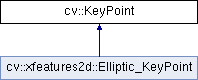OpenCV  4.1.1 Open Source Computer Vision
cv::KeyPoint Class Reference

Data structure for salient point detectors. More...

#include <opencv2/core/types.hpp>

Inheritance diagram for cv::KeyPoint:## Public Member Functions

KeyPoint ()
the default constructor More...

KeyPoint (Point2f _pt, float _size, float _angle=-1, float _response=0, int _octave=0, int _class_id=-1)

KeyPoint (float x, float y, float _size, float _angle=-1, float _response=0, int _octave=0, int _class_id=-1)

size_t hash () const

## Static Public Member Functions

static void convert (const std::vector< KeyPoint > &keypoints, std::vector< Point2f > &points2f, const std::vector< int > &keypointIndexes=std::vector< int >())

static void convert (const std::vector< Point2f > &points2f, std::vector< KeyPoint > &keypoints, float size=1, float response=1, int octave=0, int class_id=-1)

static float overlap (const KeyPoint &kp1, const KeyPoint &kp2)

## Public Attributes

float angle

int class_id
object class (if the keypoints need to be clustered by an object they belong to) More...

int octave
octave (pyramid layer) from which the keypoint has been extracted More...

Point2f pt
coordinates of the keypoints More...

float response
the response by which the most strong keypoints have been selected. Can be used for the further sorting or subsampling More...

float size
diameter of the meaningful keypoint neighborhood More...

## Detailed Description

Data structure for salient point detectors.

The class instance stores a keypoint, i.e. a point feature found by one of many available keypoint detectors, such as Harris corner detector, FAST, StarDetector, SURF, SIFT etc.

The keypoint is characterized by the 2D position, scale (proportional to the diameter of the neighborhood that needs to be taken into account), orientation and some other parameters. The keypoint neighborhood is then analyzed by another algorithm that builds a descriptor (usually represented as a feature vector). The keypoints representing the same object in different images can then be matched using KDTree or another method.

## § KeyPoint() [1/3]

 cv::KeyPoint::KeyPoint ( )
Python:
<KeyPoint object>=cv.KeyPoint()
<KeyPoint object>=cv.KeyPoint(x, y, _size[, _angle[, _response[, _octave[, _class_id]]]])

the default constructor

## § KeyPoint() [2/3]

 cv::KeyPoint::KeyPoint ( Point2f _pt, float _size, float _angle = -1, float _response = 0, int _octave = 0, int _class_id = -1 )
Python:
<KeyPoint object>=cv.KeyPoint()
<KeyPoint object>=cv.KeyPoint(x, y, _size[, _angle[, _response[, _octave[, _class_id]]]])
Parameters
 _pt x & y coordinates of the keypoint _size keypoint diameter _angle keypoint orientation _response keypoint detector response on the keypoint (that is, strength of the keypoint) _octave pyramid octave in which the keypoint has been detected _class_id object id

## § KeyPoint() [3/3]

 cv::KeyPoint::KeyPoint ( float x, float y, float _size, float _angle = -1, float _response = 0, int _octave = 0, int _class_id = -1 )
Python:
<KeyPoint object>=cv.KeyPoint()
<KeyPoint object>=cv.KeyPoint(x, y, _size[, _angle[, _response[, _octave[, _class_id]]]])
Parameters
 x x-coordinate of the keypoint y y-coordinate of the keypoint _size keypoint diameter _angle keypoint orientation _response keypoint detector response on the keypoint (that is, strength of the keypoint) _octave pyramid octave in which the keypoint has been detected _class_id object id

## § convert() [1/2]

 static void cv::KeyPoint::convert ( const std::vector< KeyPoint > & keypoints, std::vector< Point2f > & points2f, const std::vector< int > & keypointIndexes = std::vector< int >() )
static
Python:
points2f=cv.KeyPoint_convert(keypoints[, keypointIndexes])
keypoints=cv.KeyPoint_convert(points2f[, size[, response[, octave[, class_id]]]])

This method converts vector of keypoints to vector of points or the reverse, where each keypoint is assigned the same size and the same orientation.

Parameters
 keypoints Keypoints obtained from any feature detection algorithm like SIFT/SURF/ORB points2f Array of (x,y) coordinates of each keypoint keypointIndexes Array of indexes of keypoints to be converted to points. (Acts like a mask to convert only specified keypoints)

## § convert() [2/2]

 static void cv::KeyPoint::convert ( const std::vector< Point2f > & points2f, std::vector< KeyPoint > & keypoints, float size = 1, float response = 1, int octave = 0, int class_id = -1 )
static
Python:
points2f=cv.KeyPoint_convert(keypoints[, keypointIndexes])
keypoints=cv.KeyPoint_convert(points2f[, size[, response[, octave[, class_id]]]])

This is an overloaded member function, provided for convenience. It differs from the above function only in what argument(s) it accepts.

Parameters
 points2f Array of (x,y) coordinates of each keypoint keypoints Keypoints obtained from any feature detection algorithm like SIFT/SURF/ORB size keypoint diameter response keypoint detector response on the keypoint (that is, strength of the keypoint) octave pyramid octave in which the keypoint has been detected class_id object id

## § hash()

 size_t cv::KeyPoint::hash ( ) const

## § overlap()

 static float cv::KeyPoint::overlap ( const KeyPoint & kp1, const KeyPoint & kp2 )
static
Python:
retval=cv.KeyPoint_overlap(kp1, kp2)

This method computes overlap for pair of keypoints. Overlap is the ratio between area of keypoint regions' intersection and area of keypoint regions' union (considering keypoint region as circle). If they don't overlap, we get zero. If they coincide at same location with same size, we get 1.

Parameters
 kp1 First keypoint kp2 Second keypoint

## § angle

 float cv::KeyPoint::angle

computed orientation of the keypoint (-1 if not applicable); it's in [0,360) degrees and measured relative to image coordinate system, ie in clockwise.

## § class_id

 int cv::KeyPoint::class_id

object class (if the keypoints need to be clustered by an object they belong to)

## § octave

 int cv::KeyPoint::octave

octave (pyramid layer) from which the keypoint has been extracted

## § pt

 Point2f cv::KeyPoint::pt

coordinates of the keypoints

## § response

 float cv::KeyPoint::response

the response by which the most strong keypoints have been selected. Can be used for the further sorting or subsampling

## § size

 float cv::KeyPoint::size

diameter of the meaningful keypoint neighborhood

The documentation for this class was generated from the following file: